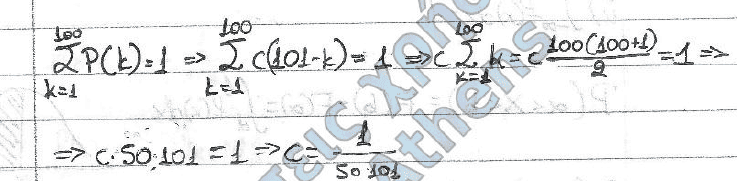# Probabilities question

That's not exactly coursework (it's studying) but I've spammed the probabilities forum too much.

Can someone explain this part?

Knowing that P(k)=P(X=k)=c(101-k)

For a 'turtle' that can live up to 100 years, find 'c'.

And it goesI don't understand the jump from cΣk to c100(100+1)/2

$$\sum_{k=1}^n k = (1+n) \frac{n}{2}$$